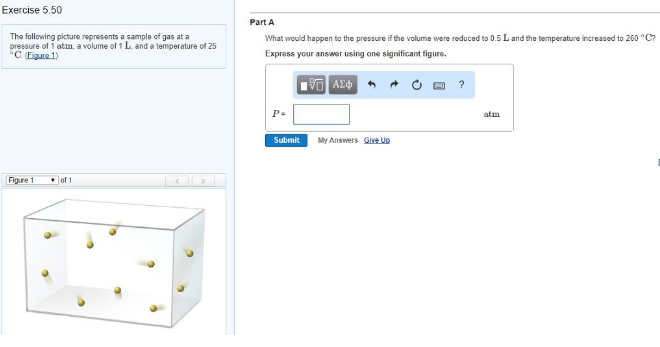# Problem: The following picture represents a sample of gas at a pressure of 1 atm, a volume of 1 L, and a temperature of 25°C. (Figure 1)What would happen to the pressure if the volume were reduced to 0.5 L and the temperature increased to 260°C? Express your answer using one significant figure.

###### FREE Expert Solution
95% (59 ratings)###### Problem Details

The following picture represents a sample of gas at a pressure of 1 atm, a volume of 1 L, and a temperature of 25°C. (Figure 1)

What would happen to the pressure if the volume were reduced to 0.5 L and the temperature increased to 260°C?